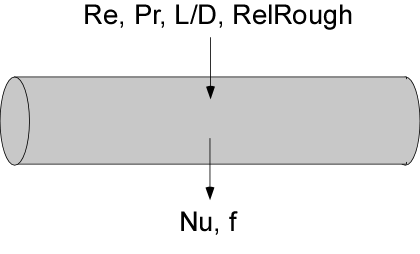Contents - Index

PipeFlow_NDProcedure PipeFlow_ND(Re, Pr, LoverD, RelRough: Nusselt_T, Nusselt_H, f) returns the average Nusselt number assuming constant temperature wall and constant heat flux for a given flow condition in a circular tube characterized by Reynolds and Prandtl numbers, length/diameter, and relative roughness.  The Procedure also returns the friction factor for these conditions.   The procedure can be used for developing or fully-developed flow in the laminar, transitional or turbulent flow regimes.

Inputs:

Re - Reynolds number based on properties evaluated at the bulk average temperature

Pr - Prandtl number of the fluid evaluated at the bulk average temperature

LoverD - ratio of tube length to diameter.

RelRough - the ratio of the dispersions on the wall of the tube to the tube diameter (must be between 0 and 0.05)

Outputs:

Nusselt_T - average Nusselt number assuming a constant wall temperature

Nusselt_H - average Nusselt number assuming a constant wall heat flux

f -  apparent friction factor [-]

Notes

This procedure  is called by  PipeFlow.  It calls PipeFlow_Laminar and PipeFlow_Turbulent to obtain the appropriate Nusselt numbers and friction factor.

The procedure will determine if the flow is laminar or turbulent.  Transitional flow is assumed to occur for Reynold's numbers between 2300 and 3000 and interpolation is applied between the laminar and turbulent correlations.

The ratio LoverD is used to apply a developing flow correction based on simultaneous thermal and hydrodynamic development.  Set LoverD to a large number if developing flow corrections are not applicable.  Set the Prandtl number to a large value to obtain results that are consistent with thermally developing/hydrodynamically developed conditions for laminar flows.

Two Nusselt numbers are returned.  Nusselt_T corresponds to a constant temperature wall and Nusselt_H corresponds to a constant heat flux.  These two values will be the same for turbulent flow.  For laminar flow, they provide upper and lower bounds on the Nusselt number as explained in Section 5.2.4 of Nellis and Klein.

Example

Re=10000

Pr=2

LoverD=100

relRough=0

call PipeFlow_ND(Re,Pr,LoverD,RelRough: Nusselt_T,Nusselt_H,f)

{Solution

f=0.03211 [-]

Nusselt_H=49.37

Nusselt_T=49.37 [-]}